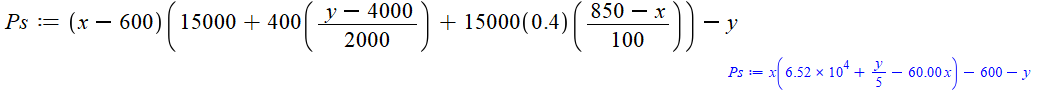## Range of parameter in NonlinearFit...

Hi,

I'd like to know, if it is possible to define any sort of range for parameters in NonlinearFit. E. g. I know that one of parameters should be somewhere between 0.2 - 0.4. I know there is a possibility of initalvalues, but using it doesn't lead into this range.

Thanks.

## Square brackets...

Hi. So my question is how can I get Square brackets on the phone app in calculations or is it possible?

## Do you have any idea how to solve this problem?...

In a french magazine written by High Schools teachers I found this problem:

```let a, b, p, q four strictly positive integers such that a > b^2 and p > q+1;
find 4-tuples (a, b, p, q) such that

(a^2 - b^4) = p!/q!```

Given the source of this problem I suspect that there is a trick to answering this question.
After some hours spent, I have found no general method to solve it, only a few solutions (first one and second one are almost obvious), for instance

```rel := a^2 - b^4 = p!/q!:

eval(rel, [a= 5, b=1, q=1, p=4]);
eval(rel, [a=11, b=1, q=1, p=5]);
eval(rel, [a=71, b=1, q=1, p=7]);
eval(rel, [a= 2, b=1, q=2, p=3]);
eval(rel, [a=19, b=1, q=2, p=6]);
eval(rel, [a=21, b=3, q=2, p=6]);

```

Do you have any idea how to solve this problem?
Could it be handled by Maple (without a systematic exploration of a part of N^4)?

## slope angle for a line or lines in plot?...

lets say we have one or two lines

y=2x-4 and y = -2x+4 is it possible to get Maple to illustrate the angle between these two lines in a plot? Or the angle of inclination in respect to the x-axes for them individually?

## How to get the Laplace inverse of this function? (...

kindly help me to find the inverse Laplace of this function. I tried but maple leaves the integral unevaluated.

 >>>(1)
 >(2)
 >## revision of error ...

how can revision of error in

restart;
u0 := proc (x) options operator, arrow; 1+2*x end proc; h := 0;
for k from 0 to 5 do U := proc (k, h) options operator, arrow; eval((diff(u0(x), [`\$`(x, k)]))/factorial(k), x = 0) end proc end do;
m := h+1;
for k from 0 to 5 do U := proc (k, h) options operator, arrow; (sum(sum(U(r, h-s)*U(k-r, s), s = 0 .. h), r = 0 .. k)+(k+1)*U(k, h))/m end proc end do;
U(0, 1);
Error, (in U) too many levels of recursion

## Why is Maple incorrectly simplifying my expression...

I have the following expression.Maple will evaluate this to:Screenshot:Plotting these two in 2D on Desmos to demonstrate: https://www.desmos.com/calculator/tvp4rbzxzp

These two are not the same expression. Is Maple broken or am I doing something wrong?

## Solution of the ODE system...

 >>>(1)
 >>(2)
 >>